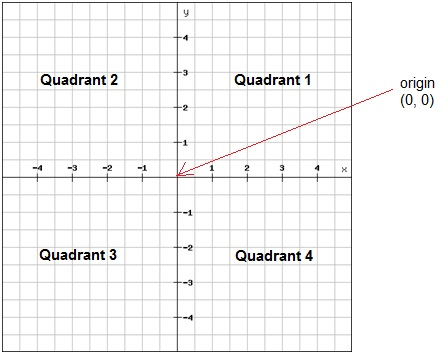# The coordinate plane

As you remember from pre-algebra a coordinate plane is a two-dimensional number line where the vertical line is called the y-axis and the horizontal is called the x-axis. These lines are perpendicular and intersect at their zero points. This point is called the origin. The axes divide the plane into four quadrants.A point in a coordinate plane is named by its ordered pair of the form of (x, y). The first number corresponds to the x-coordinates and the second to the y-coordinate.

The completeness property for points in the plane tells us two things

1. Exactly one point in the plane is named given the numbers of the ordered pair and
2. Exactly one ordered pair of numbers names a given point in the plane.

To graph a point one draws a dot at the coordinates that corresponds to the ordered pair. It's always a good idea to start at the origin. The x-coordinate tells you have many steps you have to take to the right (positive) or left (negative). And the y-coordinate tells you have many steps to move up (positive) or down (negative).

To find out the coordinates of a point in the coordinate system you do the opposite. Begin at the point and follow a vertical line either up or down to the x-axis. There is your x-coordinate. And then do the same but following a horizontal line to find the y-coordinate.

A relation is a set of ordered pairs. The first coordinate (usually the x-coordinate) is called the domain and the second (usually the y-coordinate) is called the range. If you remember from earlier chapters the domain contains values that correspond to the independent variable whereas the range contains values corresponding to the dependent variable.

## Video lesson

Draw the following ordered pairs in the coordinate plane

(0, 0); (0,4); (4, -2); (-2, -4); (1, 3)